In the previous section, we have learned about one dimensional pattern of digital roots. In this section, we will explore the properties of simple arithmetic of digital roots. From these operations, we can derive two dimensional pattern of digital root.

Suppose we have two digital roots,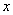and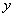, then we would like to know know the results of the arithmetic operation of digital root such as

• Addition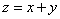• Subtraction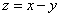• Multiplication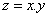• Division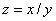Let us define digital root as a function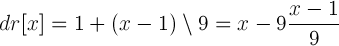, then for all the four operations above, we can say that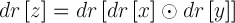## AdditionDigital root of a summation is equal to the digital root of the sum of the digital roots of each term: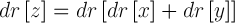For instance, we know that 589 = 214 + 375. Then, we can say that the digital root of 589 is equal to digital root of 214 plus digital root of 375. This gives digital root of 7+6 which is equal to 4. In formula, it becomes
dr = dr [ dr + dr ] = dr[7 + 6] = 4.

Here is the addition table of digital roots and its pattern.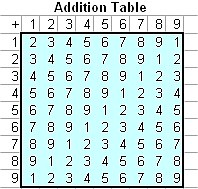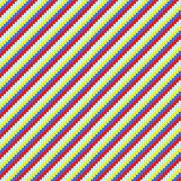## SubtractionDigital root of a difference is equal to the digital root of the difference of the digital roots of each term: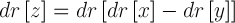For instance, we know that 161 = 375-214. Then, we can say that the digital root of 161 is equal to digital root of the difference of digital root of 375 and digital root of 214, that is equal to digital root of 6 - 7 equal to 8. In formula, it becomes

dr = dr[ dr - dr ] = dr[6-7] = 8.

Below is the Subtraction table of digital roots and its pattern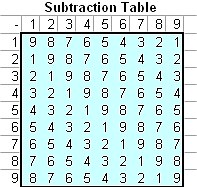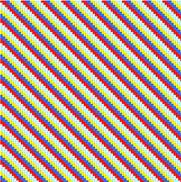You will see number 9 in the main diagonal and the other digital roots lie in parallel with the diagonal.

## MultiplicationDigital root of a product is the same as the digital root of the multiplication of the digital roots of its factors: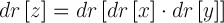For instance, we know that 7777 = 77 * 101. Then, the digital root of 7777 is equal to digital root of 5 multiply by digital root of 2, that is the digital root of 10, which is equal to 1. In formula, it becomes

dr = dr[ dr . dr ] = dr[5.2] = dr = 1.

Below is the Multiplication table of digital roots and its pattern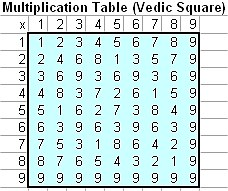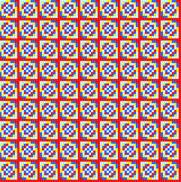Multiplication table produces many beautiful pattern and properties that worth to put into separate section to describe about it. More about multiplication table, see Vedic Square

## DivisionDigital root of a division is equal to the digital root of the difference of the digital roots of each term: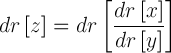For instance, we know that 31 = 3751/121. Then, we can say that the digital root of 31 is equal to digital root of the division of digital root of 3751 and digital root of 121, that is equal to digital root of 7/4 equal to 4. In formula, it becomes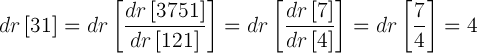ee the division table below to obtain the digital root of division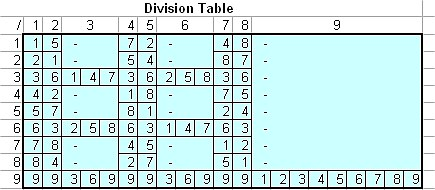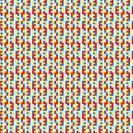Digital root 3, 6 and 9 are special. Not all numbers can be divided by these three numbers. If the division is allowed, it will produce multiple roots.

Notice that 3, 6 and 9 has no root or multiple roots. For example, 1/3 has no root (undefined, similar to a number divided by zero in decimal system) but 6/3 has multiple roots of 2, 5 and 8. Digital root 9 has no root unless the denominator is also 9 which produce 9 multiple roots of 1, 2, 3, 4, 5, 6, 7, 8, and 9.

## Factors

Suppose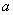,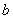andare digital roots and we have equation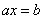then we have solution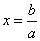1. no solution (no root) if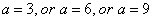and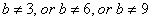2. multiple solutions (multiple roots) ifand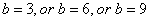3. Single solution (single root) if otherwise

## Digital Root Identity

In multiplication and division operations, digital root 1 serves as identity because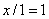and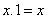. In addition or subtraction operations, digital root 9 serves as identity because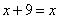and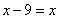. In other words, digital root 9 behaves similar to zero in decimal number system.

Preferable reference for this tutorial is

Teknomo, Kardi (2005). Digital Root. https:\\people.revoledu.com\kardi\tutorial\DigitSum\